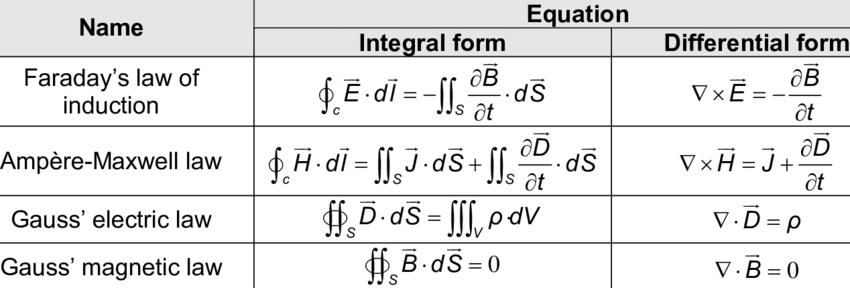# Speed of Light - A Universal Constant, General Background#### Image credit © Flicker/ Paulius Malinovskis

During the beginning of 17th century, almost all consider light as it does not have velocity. In the early period, many also assumed that the light travels instantaneously and it travels at a very fast speed. But Ole Romer stands out in these. In 1676, Danish astronomer Ole Rømer observed the eclipse time of one of Jupiter’s moon, ‘Io’ for almost a year and he encountered a strange phenomenon of that eclipse.

According to Ole Romer, this anomalous behavior of Jupiter's moon was because the light took a longer time to travel from Jupiter's moon Io and the Earth has traveled a certain distance over that course. Romer even calculated the speed of light which was about 220,000,000 m/second which on comparing with today's value - 300,000,000 m/second, is a good evaluation. Ole Romer state that the value of planet sizes he used was not all accurate. Although specifying the speed of light was a great achievement, Ole was not concerned about the reference frame and its effect on his calculation. This was assumed as the first calculation of the speed of light.

One interesting fact is that the exact coordinates of the Great Pyramid of Giza are 29.9792458°N and this number is exactly the same as the speed of light which travels at 299,792,458 metres per second.

During the mid 1800s, James Clerk Maxwell introduced Maxwell equations to measure the electric and magnetic fields in a vacuum, and that further inlight on the speed of light. By his electromagnetic equations, Maxwell claimed that the speed of massless electromagnetic radiation is very close to the supposed speed of light and further focus that they might match. Maxwell also confirmed that the light is itself an electromagnetic wave and this advancement was taken by Albert Einstein for his theory of special relativity.Different forms of Maxwell's equation. Image credit: Research Gate/ Luigi Sambuelli

For the special theory of relativity, Einstein postulated two important theory which is still valid and can't be contradicted. Among these, one prediction deals with the speed of light. Einstein's special theory contradicts Newton’s law which states that velocities add like simple vectors. Newton’s law of velocity addition in the case of speed of light fails. For example, two observers with different speeds must see the light traveling at different speeds which in reality is not viable. Also, Einstein imagined the case where an observer (person) is travelling at the speed of light and think what it would look like. He stated that if such motion exists then the light wave would be stationary relative to that observer. This will further raise a condition where electric and magnetic fields varied in strength at various distances from the observer but constant in time. This condition is fairly not allowed by Maxwell’s equations. So, Einstein concluded that either approach and conclusion of Maxwell’s equations are wrong or an object having a certain mass can never cross the speed of light. Einstein can not contradict Maxwell’s electromagnetic equation for which all had agreed and concluded that the speed of light can not be achieved by an object having mass. This further implies that light must always travel with constant speed c relative to an observer. This is further supported by Michelson-Morley experiment.

Though the speed of light is a universal constant, they are affected by the gravitational pull.

During the years of 1800, it is believed that like sound and other waves propagate through a medium and so the light must also need some medium to propagate. To experimentally verify that light travels through a medium, called ether medium, Michelson and Morley set up an experiment. They use to observe the relative changes in the speed of light as the Earth changes its direction relative to the Sun. But unfortunately, they failed to detect any changes in the speed of light.

Mathematically, the speed of light can be calculated by the definition of Planck Time - the unit of time, and Planck Length in a vacuum. Here, Planck Length divided by Planck time is equal to 299,792,422.79 m/second where the value of Planck Time is 10-43 seconds and Planck Length is 1.616255×10-35 m.